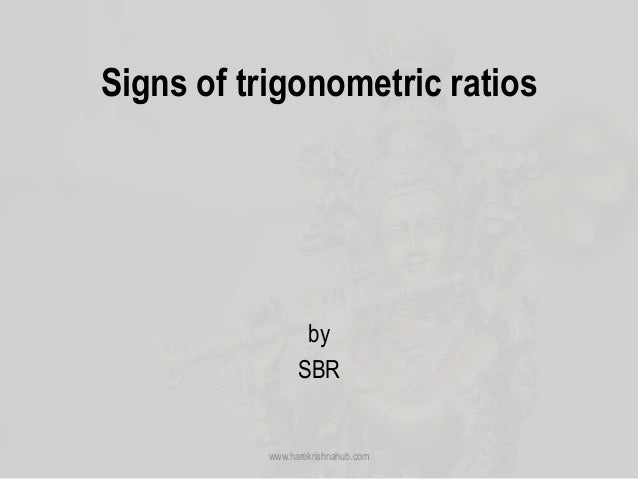Successfully reported this slideshow.
We use your LinkedIn profile and activity data to personalize ads and to show you more relevant ads. You can change your ad preferences anytime.Upcoming SlideShare
×

Signs of trigonometric ratios

10 views

Published on

This presentation illustrate the use of signs of trigonometric ratios

Published in: Education
• Full Name
Comment goes here.

Are you sure you want to Yes No
Your message goes here• Be the first to comment

• Be the first to like this

Signs of trigonometric ratios

1. 1. Signs of trigonometric ratios by SBR www.harekrishnahub.com
2. 2. www.harekrishnahub.com Consider a circle with centre 𝑶 and radius 𝒓 units. Let the circle cut the 𝒙 − 𝒂𝒙𝒊𝒔 at 𝑨 and 𝑨′ and 𝒚 − 𝒂𝒙𝒊𝒔 at 𝑩 and 𝑩′. Let 𝑷 (𝒙, 𝒚) be any point on the circumference of the circle. Join 𝑶𝑷. Let the radius vector 𝑶𝑷 make an angle 𝜽 with the positive 𝒙 − 𝒂𝒙𝒊𝒔. ∴ ∠𝑿𝑶𝑷 = 𝜽 and 𝑶𝑷 = 𝒓 Draw 𝑷𝑴 ⟂ to the 𝒙 − 𝒂𝒙𝒊𝒔. ∴ 𝑶𝑴 = 𝒙 and 𝑷𝑴 = 𝒚
3. 3. www.harekrishnahub.com ∴ we have, Points Coordinates 𝑃 𝑥, 𝑦 𝑀 𝑥, 0 𝐴 𝑟, 0 𝐵 0, 𝑟 𝐴′ −𝑟, 0 𝐵′ 0, −𝑟
4. 4. www.harekrishnahub.com ⊿𝑷𝑴𝑶 form a right angle triangle. We have, 𝒔𝒊𝒏 𝜽 = 𝒚 𝒓 𝒄𝒐 𝒔 𝜽 = 𝒙 𝒓 𝒕𝒂𝒏 𝜽 = 𝒚 𝒙 𝒓 represents the magnitude (length) of the radius vector 𝑶𝑷. It is always positive. 𝑶𝑴 and 𝑷𝑴 represents the horizontal and vertical displacement components of point 𝑷. Hence, they can be positive or negative depending the quadrant in which the radius vector 𝑶𝑷 lies.
5. 5. www.harekrishnahub.com Quadran t 𝛉 Sign Trigonometric ratios Remarks 𝑥 𝑦 𝑠𝑖𝑛 𝜃 = 𝑦 𝑟 𝑐𝑜𝑠 𝜃 = 𝑥 𝑟 𝑡𝑎𝑛 𝜃 = 𝑦 𝑥 I 0 < 𝜃 < 90 + + + + = + 𝑣𝑒 + + = + 𝑣𝑒 + + = + 𝑣𝑒 ALL +ve II 90 < 𝜃 < 180 - + + + = + 𝑣𝑒 − + = − 𝑣𝑒 + − = − 𝑣𝑒 SIN +ve III 180 < 𝜃 < 270 - - − + = − 𝑣𝑒 − + = − 𝑣𝑒 − − = + 𝑣𝑒 TAN +ve IV 270 < 𝜃 < 360 + - − + = − 𝑣𝑒 + + = + 𝑣𝑒 − + = − 𝑣𝑒 COS +ve
6. 6. www.harekrishnahub.com • Thus, in the first quadrant, all of the trigonometric ratios are positive. • In the second quadrant, only 𝒔𝒊𝒏 𝜽 and 𝒄𝒐𝒔𝒆𝒄 𝜽 are positive and all other ratios are negative. • In the third quadrant, only tan 𝜽 and cot 𝜽 are positive and all other ratios are negative. • In the fourth quadrant, only cos 𝜽 and sec 𝜽 are positive and all other ratios are negative.
7. 7. www.harekrishnahub.com The following figure is useful in remembering the signs of the trigonometric ratios.
8. 8. www.harekrishnahub.com When P Coincides with 𝑥 𝑦 𝑠𝑖𝑛 𝜃 𝑦 𝑟 𝑐𝑜𝑠 𝜃 𝑥 𝑟 𝑡𝑎𝑛 𝜃 𝑦 𝑥 𝑨 (𝜽 = 𝟎) 𝑟 0 𝑠𝑖𝑛 0 = 0 𝑐𝑜𝑠 0 = 1 𝑡𝑎𝑛 0 = 0 𝑩 𝜽 = 𝟗𝟎 0 𝑟 𝑠𝑖𝑛 90 = 1 𝑐𝑜𝑠 90 = 0 𝑡𝑎𝑛 90 = ∞ 𝑨′ 𝜽 = 𝟏𝟖𝟎 −𝑟 0 𝑠𝑖𝑛 180 = 0 𝑐𝑜𝑠 180 = −1 𝑡𝑎𝑛 180 = 0 𝑩′ 𝜽 = 𝟐𝟕𝟎 0 −𝑟 𝑠𝑖𝑛 270 = −1 𝑐𝑜𝑠 270 = 0 𝑡𝑎𝑛 270 = −∞